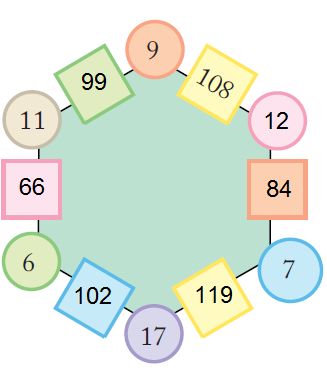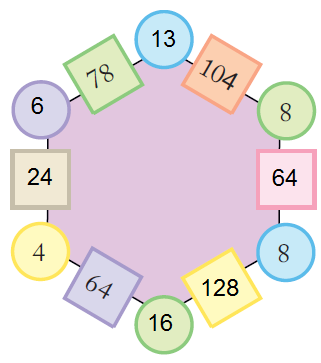# NCERT Solutions Class 5 Maths Chapter-7 Can You See The Pattern

### Can You See The Pattern

Page: 99

1.

Solution :-

Solution :-

2. What should come next?

(a)

Solution:-

(b)

Solution:-

(c)

Solution:-

(d)

Solution:-

3. See this pattern

(a)

Solution:-

4. Using the same rule take it forward till you get back to what you started with.

(a)

Solution:-

(b)

Solution:-

Solution:-

6. Mark that picture which is breaking the rule. Also correct it.

(a)

Solution:-

The below marked picture is breaking the rule.

(b)

Solution:-

The below marked picture is breaking the rule.

(c)

Solution:-

The below marked picture is breaking the rule.

(d)

Solution:-

The below marked picture is breaking the rule.

7. Magic Squares

Do you remember magic triangles? Come now, let’s make some magic squares.

(i) Fill this square using all the numbers from 46 to 54.

Rule: The total of each line is 150.

Solution:-

From the question it is give that, total of each line is equal to 150.

So, let us take third row,

From the rule, + 52 + 47 = 150

+ 99 = 150

= 150 – 99

Therefore, number in the first box in third row = 51

Now, let us take first column,

From the rule, + 46 + 51 = 150

+ 97 = 150

= 150 – 97

Therefore, number in the first box in first column = 53

Let us take first row,

From the rule, 53 + + 49 = 150

+ 102 = 150

= 150 – 102

Therefore, number in the second box in first row = 48

Let us take second column,

From the rule, 48 + + 52 = 150

+ 100 = 150

= 150 – 100

Therefore, number in the second box in second column = 50

Let us take third column,

From the rule, 49 + + 47 = 150

+ 96 = 150

= 150 – 96

Therefore, number in the second box in third column = 54

(ii) Fill this square using all the numbers from 21 to 29.

Rule: The total of each side is 75.

Solution:-

From the question it is give that, total of each line is equal to 75.

8. Magic Hexagons

Look at the patterns of numbers in hexagons. Each side has 2 circles and 1 box.

Look at the number 65 in the box. Which are the circles next to it? Can you see how the rule works?

Solution:-

The circles next to 65 are 5 and 13.

The rule of this method is we get the number in each box by multiplying the numbers in the circles next to it.

(i) Use the same rule to fill the hexagons below.

(a)Solution:-

11 × 9 = 99

11 × 6 = 66

6 × 17 = 102

17 × 7 = 119

9 × 12 = 108

12 × 7 = 84

(b)

Solution:-4 × 16 = 64

16 × 8 = 128

8 × 8 = 64

13 × 8 = 104

13 × 6 = 78

9. Numbers and Numbers

(i) Are they equal?

Solution:-

Yes, the mentioned equation are equal.

Because, let us consider left hand side (LHS) of first equation = 24 + 19 + 37

LHS = 80

Now, Right hand side (RHS) = 37 + 24 + 19

RHS = 80

By comparing LHS and RHS,

LHS = RHS

Then consider second equation, LHS = 215 + 120 + 600

LHS = 935

Now, RHS = 600 + 215 + 120

= 935

By comparing LHS and RHS,

LHS = RHS

(ii) Fill in the blank spaces in the same way.

(a)

Solution:-

(b)

Solution:-

(c)

Solution:-

(iii) Now,look at this-

Check if it is true or not.

Solution:-

First consider the left hand side (LHS) = 48 × 13

LHS = 624

Now consider right hand side (RHS) = 13 × 48

RHS = 624

By comparing LHS and RHS,

LHS = RHS

(iv) Now you try and change these numbers into special numbers

(a) 28

Solution:-

Take another number 28

Now turn it back to front 82

Is this a special number? No! Why not?

OK, carry on with the number 110

Again turn it back to front 011

Then add the two together 121

Ah! 121 is a special number.

(b) 132

Solution:-

Take another number 132

Now turn it back to front 231

Ah! 363 is a special number.

(c) 273

Solution:-

Take another number 273

Now turn it back to front 372

Is this a special number? No! Why not?

OK, carry on with the number 645

Again turn it back to front 546

Then add the two together 1191

Is this a special number? No! Why not?

OK, carry on with the number 1191

Again turn it back to front 1911

Then add the two together 3102

Is this a special number? No! Why not?

OK, carry on with the number 3102

Again turn it back to front 2013

Then add the two together 5115

Ah! 5115 is a special number.

(v) Now let’s use words in a special way.

Did you notice that it reads the same from both sides — right to left and left to right?

Solution:-

EYE, LEVEL, ROTATOR, NOON, REFER, TOP SPOT etc.

10. Some more Number Patterns

(i) Take any number. Now multiply it by 2, 3, 4 …………… at every step. Also add 3 to it at each step. Look at the difference in the answer. Is it the same at every step?

Solution:-

Let us check difference in the answer, 39 – 27 = 12, 51 – 39 = 12, 63 – 51 = 12,

75 – 63 = 12, 87 – 75 = 12, 99 – 87 = 12, 111 – 99 = 12.

Therefore, the difference in the answer are same at every step.

(ii) Look at the numbers below. Look for the pattern. Can you take it forward?

Solution:-

Solution:-

(ii) Did you notice some pattern in the answers?

Solution:-

Yes, I found that the difference in the answer are same i.e. 100 at every step.

12. Fun with Odd Numbers

Take the first two odd numbers. Now add them, see what you get. Now, at every step, add the next odd number. How far can you go on?

Solution:-

We can’t predict, because there are infinite numbers.

13. Secret Numbers

Banno and Binod were playing a guessing game by writing clues about a secret number. Each tried to guess the other’s secret number from the clues. Can you guess their secret numbers?

(i) It is larger than half of 100

It is more than 6 tens and less than 7 tens

The tens digit is one more than the ones digit

Together the digits have a sum of 11

Solution:-

It is larger than half of 100, i.e. number > 100

It is more than 6 tens and less than 7 tens = so, number lies between 70 and 60

The tens digit is one more than the ones digit = 6 – 1 = 5

Together the digits have a sum of 11 = 6 + 5 = 11

Therefore the number is 65

(ii) It is smaller than half of 100

It is more than 4 tens and less than 5 tens

The tens digit is two more than the ones digit

Together the digits have a sum of 6

Solution:-

It is smaller than half of 100 = number > 100

It is more than 4 tens and less than 5 tens = number lies between 40 and 50

The tens digit is two more than the ones digit = 4 – 2 = 2

Together the digits have a sum of 6 = 4 + 2 = 6

Therefore the number is 42

14. Number Surprises

a) Ask your friend rite — W down your age. Add 5 to it. Multiply the sum by 2. Subtract 10 from it. Next divide it by 2. What do you get? Is your friend surprised?

Solution:-

Let us assume the age be 11,

Then, adding 5 to it we get = 16

Multiply by 2 we get = 32

Subtract from 10 we get = 22

Divided by 2 we get = 11

Yes, my friend was really surprised.

Solution:-

Solution:-

Solution:-

1 = 1 × 1

121 = 11 × 11

12321 = 111 × 111

1234321 = 1111 × 1111

123454321 = 11111 × 11111

12345654321 = 111111 × 111111

1234567654321 = 1111111 × 1111111

1234567654321 = 1111111 × 1111111

1234567654321 = 1111111 × 1111111